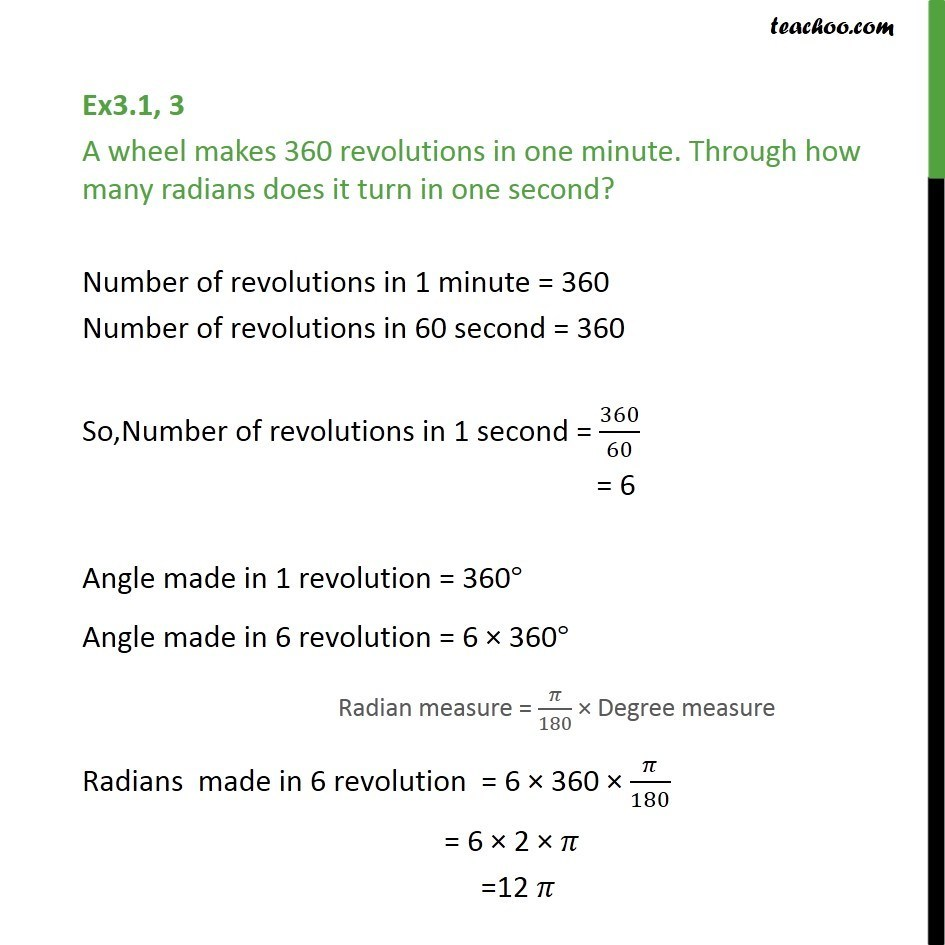1. Chapter 3 Class 11 Trigonometric Functions (Term 2)
2. Serial order wise
3. Ex 3.1

Transcript

Ex3.1, 3 A wheel makes 360 revolutions in one minute. Through how many radians does it turn in one second? Number of revolutions in 1 minute = 360 Number of revolutions in 60 second = 360 So,Number of revolutions in 1 second = 360/60 = 6 Angle made in 1 revolution = 360 Angle made in 6 revolution = 6 360 Radians made in 6 revolution = 6 360 /180 = 6 2 =12